# Forecasting

Zoho Analytics enables you to effectively predict your future data trends, using its forecasting feature. Forecasting in Zoho Analytics is based on powerful forecasting algorithms which analyze your past data deeply and come up with the best forecast for the future. You can set up a forecast in a chart using a very simple set up process, without worrying about the underlying complexity.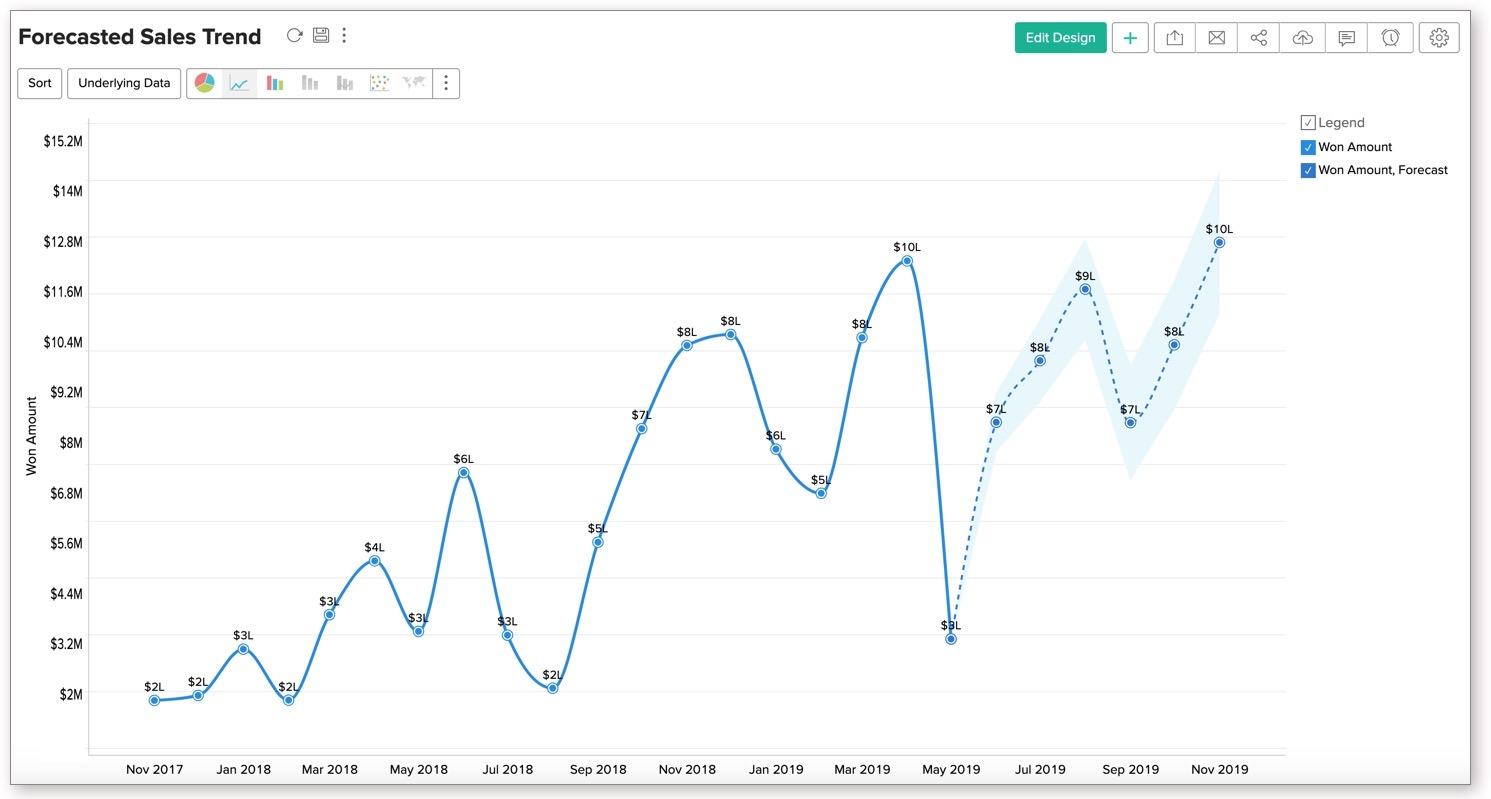This document will help you to learn how forecasting works in Zoho Analytics and how you can easily set up the same in your charts.

### 1. What is forecasting?

Forecasting is a process of predicting the future based on the past data trend. Zoho Analytics forecasting is based on powerful forecasting algorithms which analyzes your past data deeply and comes up with the best forecast for the future.

### 2. What are the chart types that support Forecasting?

Zoho Analytics supports forecasting for the following chart types.

• Line Chart
• Bar Chart
• Stacked Bar Chart
• Scatter Chart
• Area Chart
• Stacked Area Chart
• Combo Charts (without Bubble Chart).

### 4. Can I set up forecasting over What-if Analysis?

Yes, you can. Zoho Analytics What-if Analysis enables you to measure the difference in outcome when one or more variables change. Applying Forecast over a What-if metric column helps you see how the forecasted values will change.

Setting up forecast over a What-if Analysis is similar to any other chart. What-If will be applied over the entire chart, including forecasted data.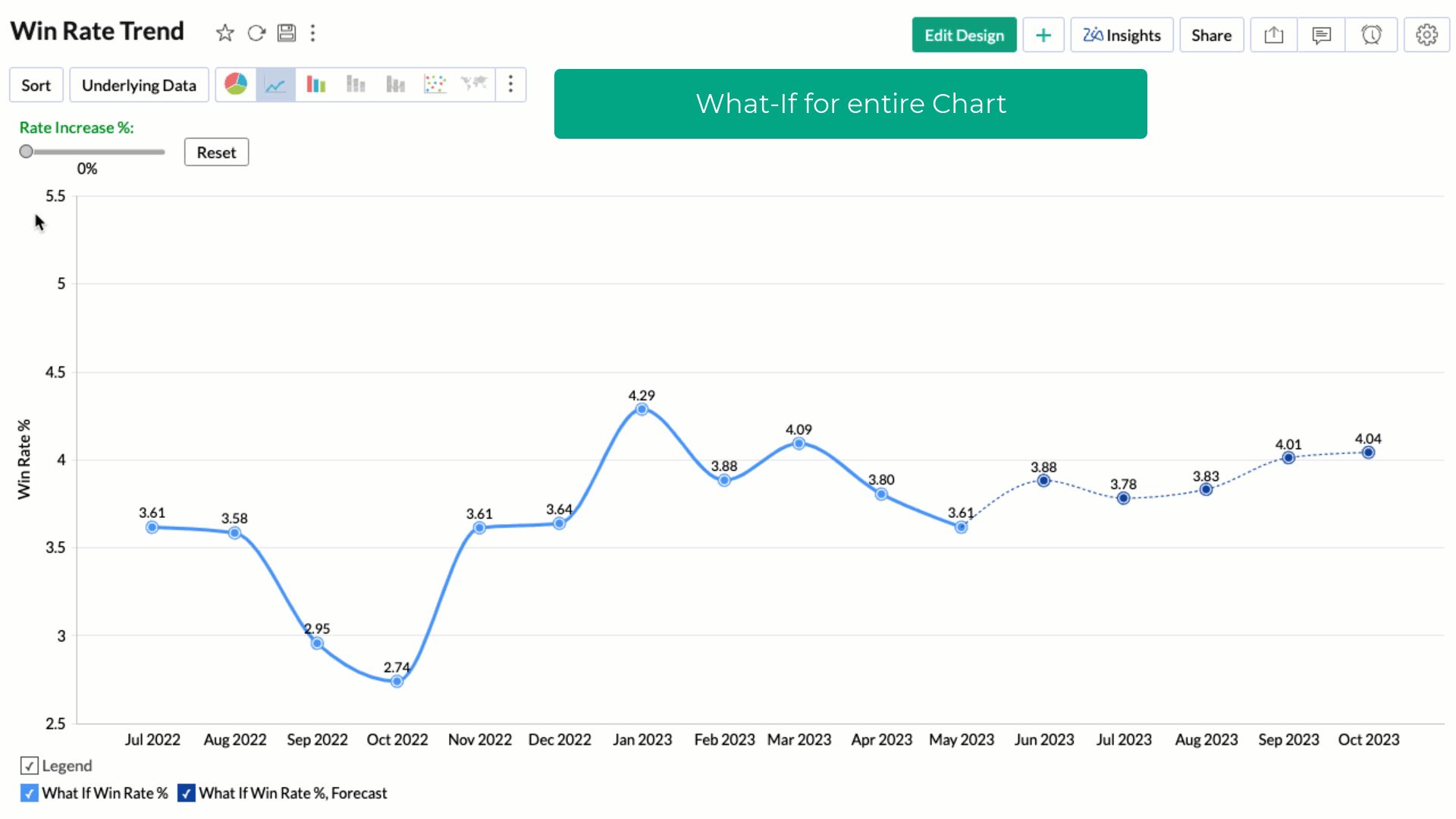You can choose to apply the What-if factor over the forecasted data alone by selecting the Treat as What-if Forecasting checkbox.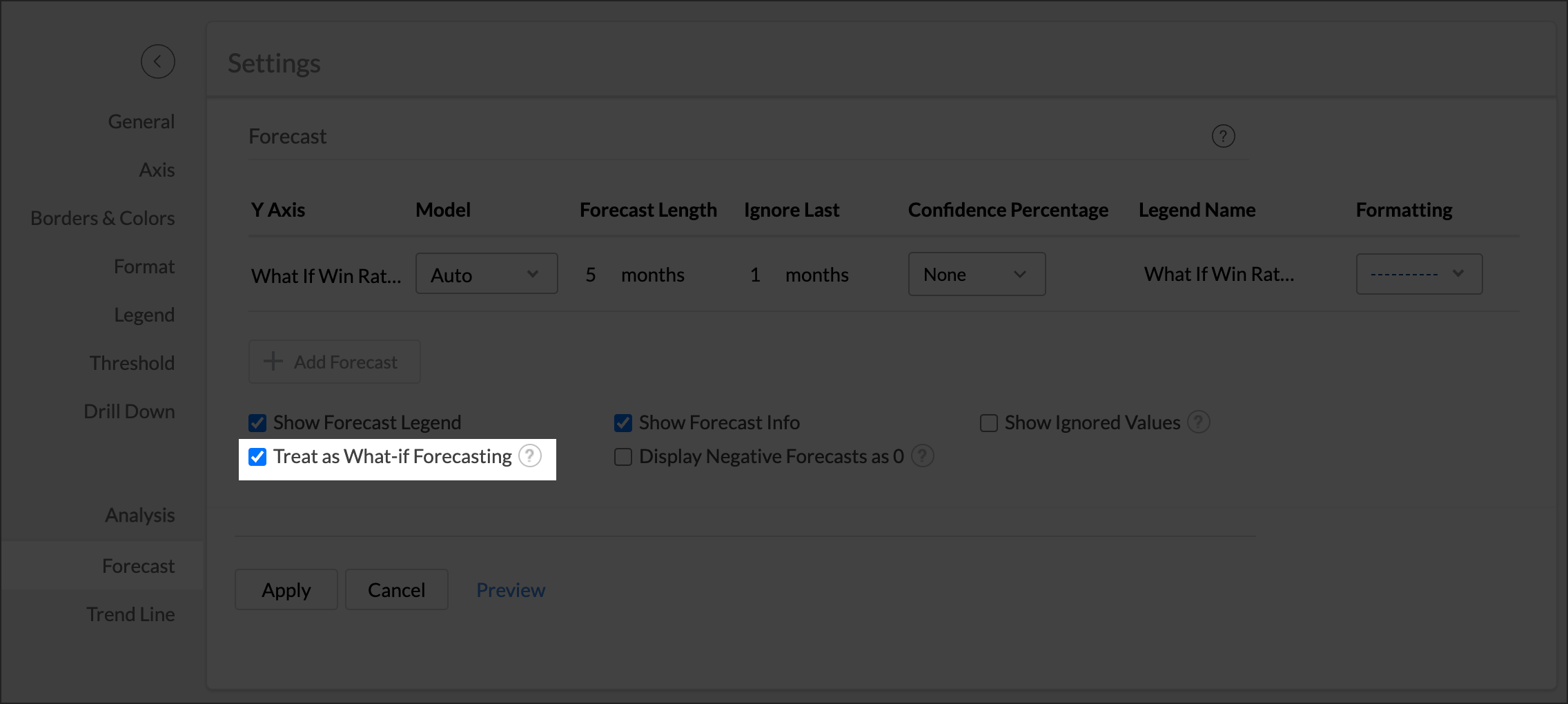The following screen illustrates What-If Analysis for forecast data alone.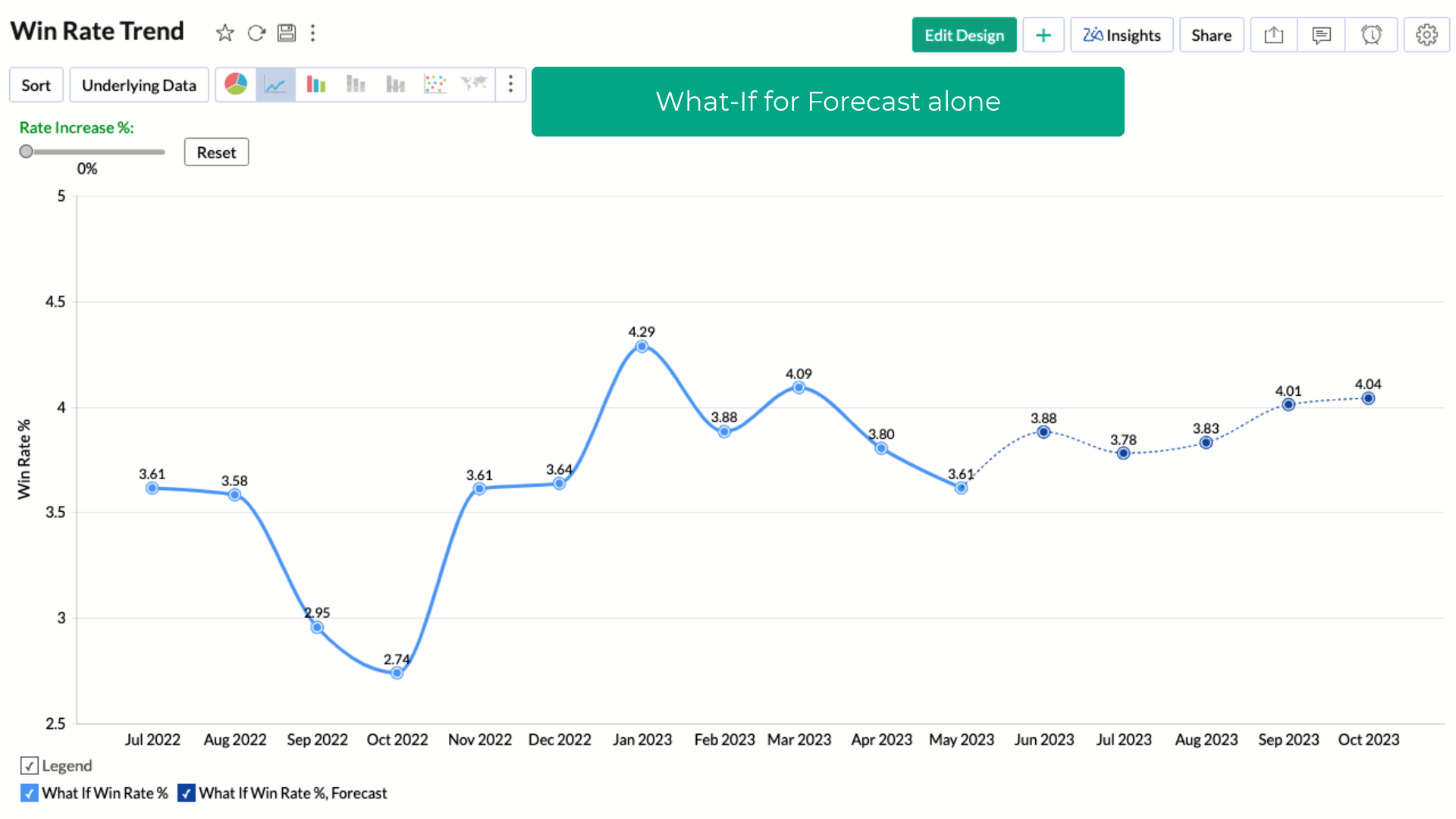### 4. Can I set up forecasting over multiple Y-Axis?

Yes, you can set up forecasting over multiple Y-Axis in a chart.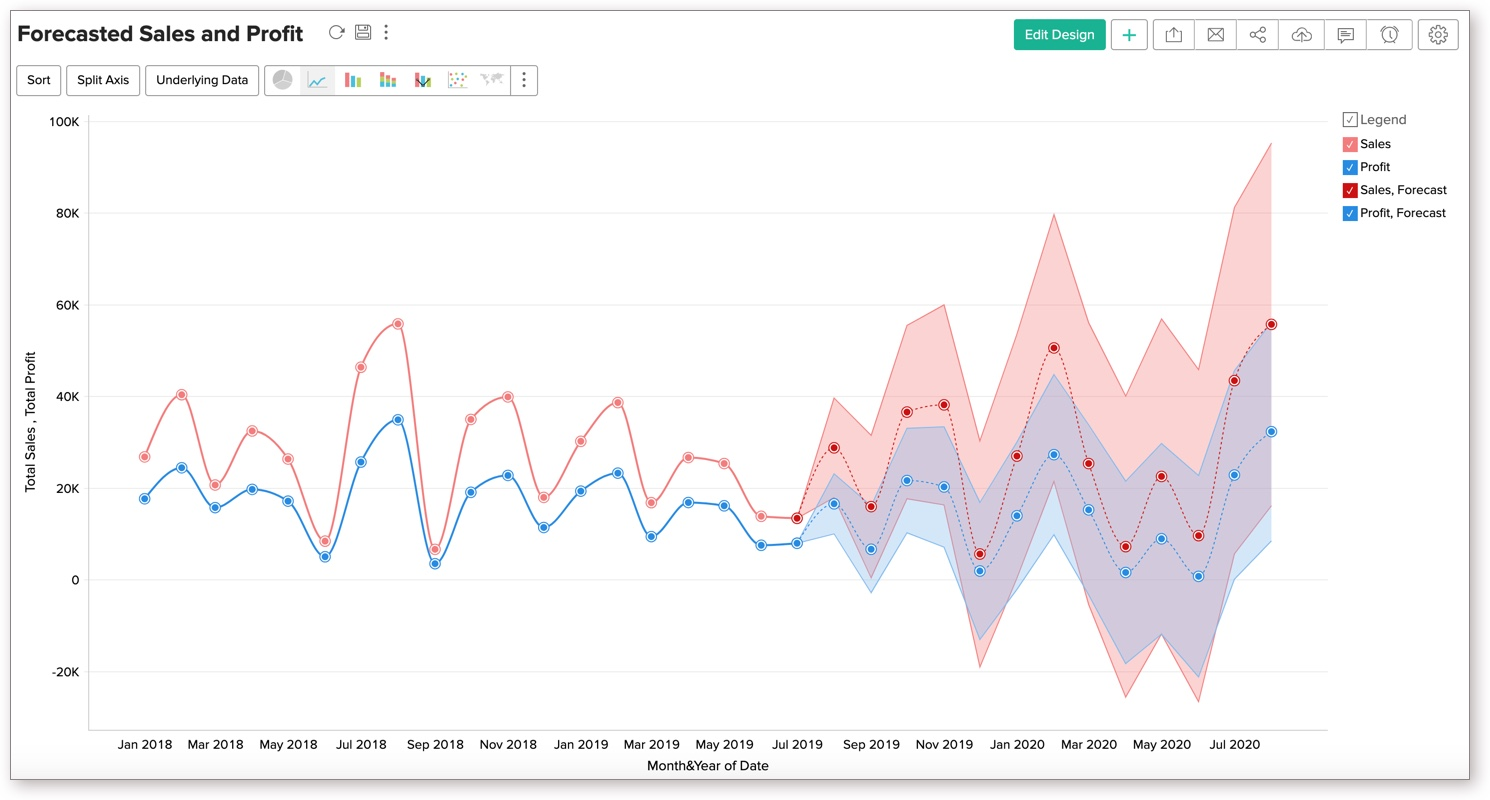### 5. Can I set up forecasting in a chart that has a column in the Color shelf?

Yes, you can set up forecasting over a chart with Color. Apply Forecasting based on Y-Axis. It will be applied for all the Color series.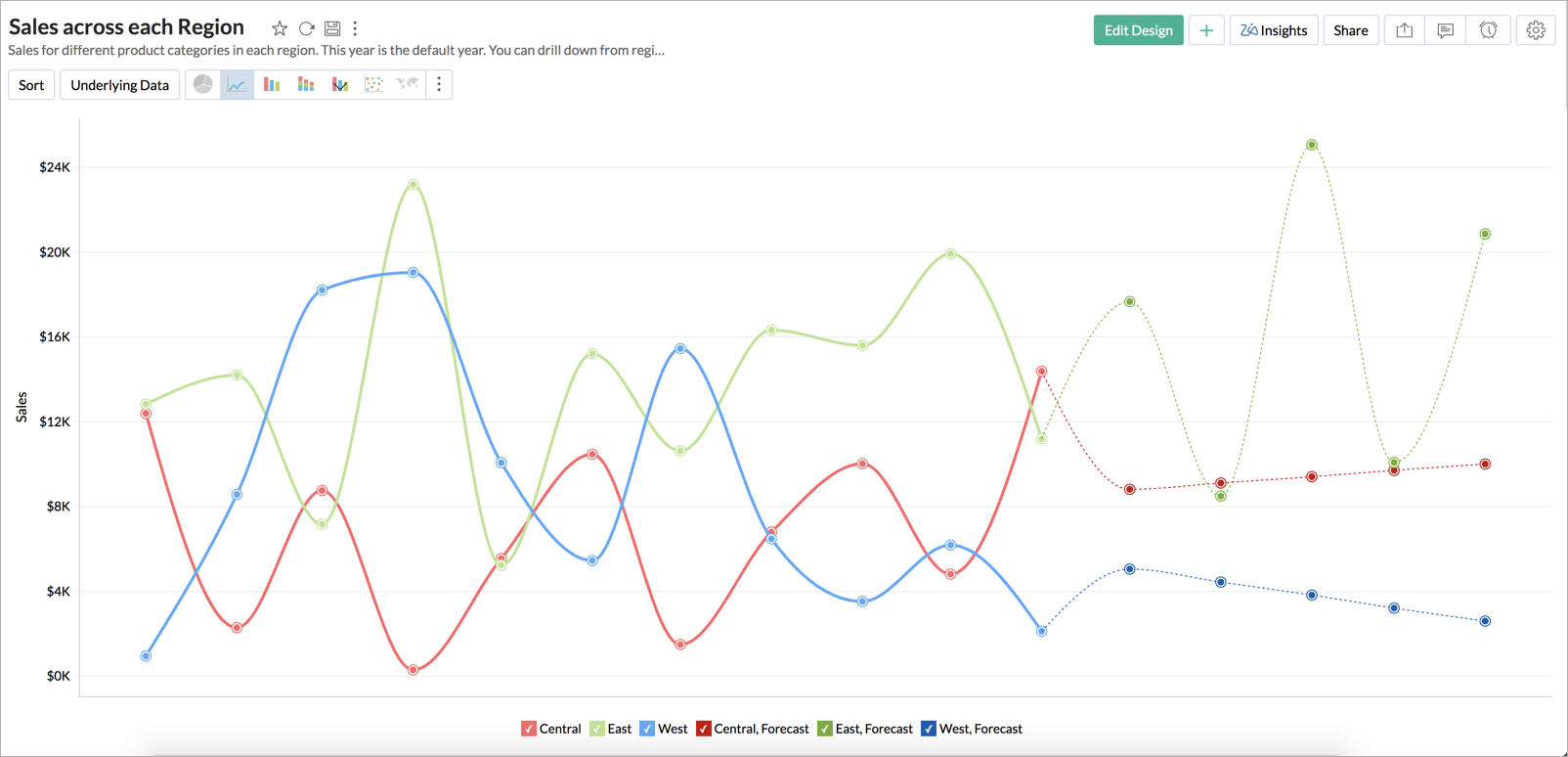### 6. Forecast option is not available in my Chart settings, why?

Forecasting will be enabled when it matches certain conditions, which are briefed below:

• The chart type should be any one of the following:
Line Chart, Bar Chart, Stacked Bar Chart, Scatter Chart, Area Chart, Stacked Area Chart, Web Chart, Combo Charts.
• The X-axis of the chart should be a TIME series or NUMBER series.
• Charts should have only one dimension column in X-axis: Forecasting will be enabled only when the chart has a single dimension column (in X-axis). If the chart contains Text, Color, Size or Tooltip, then forecasting will not be enabled.
• Atleast one aggregate function should be present in Y-axis: Forecasting can be applied over the aggregate function alone. if all the Y-axis series in the chart contain advanced summarizing options like running total, then forecasting will not be enabled.
• If the chart is filtered with a numeric column, then forecasting will be disabled. The forecasting based on filtered data might give inaccurate results, hence forecasting is disabled.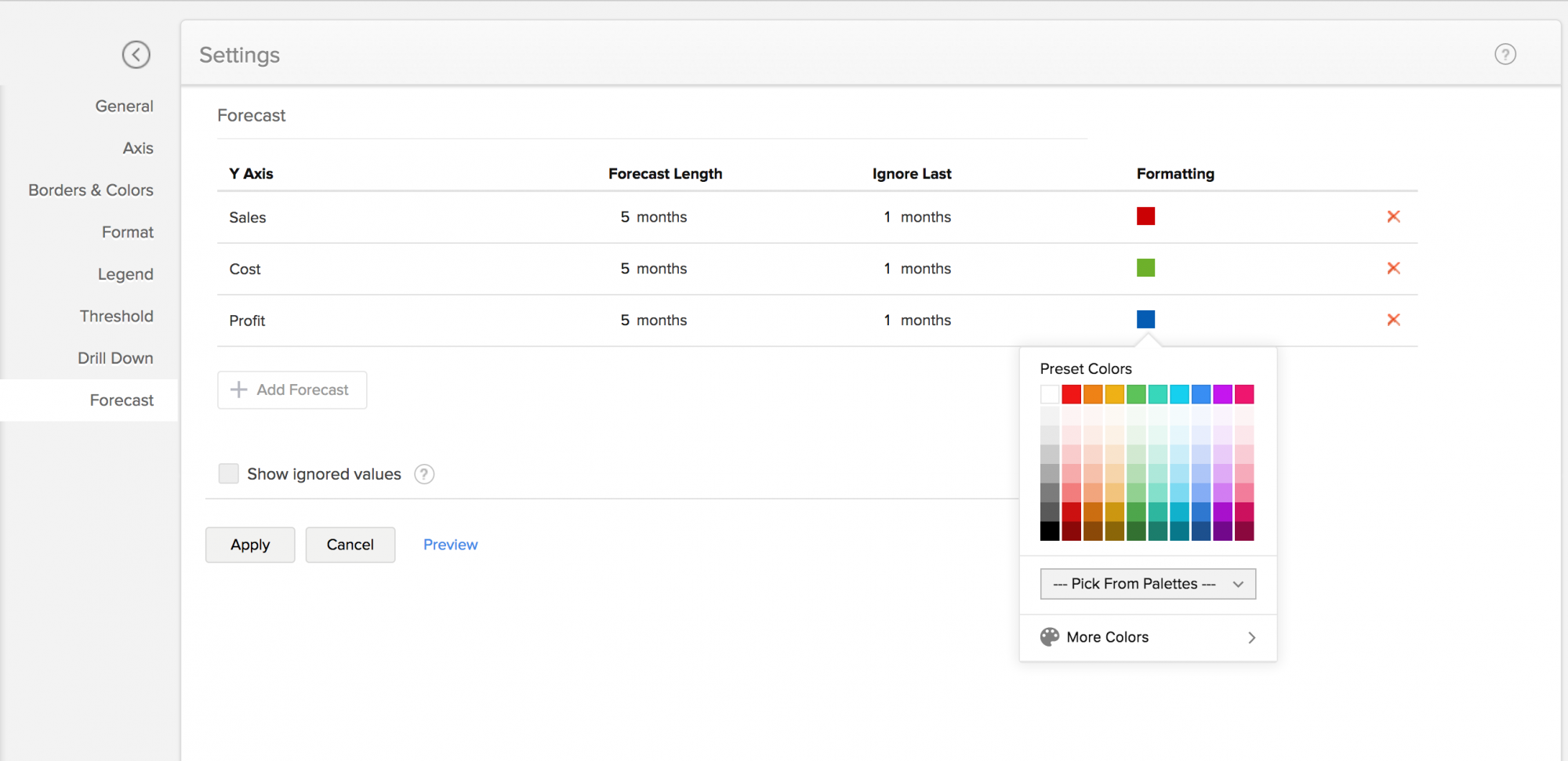### 7. How Forecasting works in Zoho Analytics?

Zoho Analytics offers a powerful forecasting engine which predicts future data points based on past data. The forecasting engine offers a range of customizations such as number of units to be forecasted, number of data points to be ignored in the past data and the formatting to be applied over the forecasted data points.

The following points describe how the forecasting engine works in Zoho Analytics:

1. The forecasting engine analyses past data points.
2. Based on the past data, the forecasting engine will identify the periodicity using auto-correlation method.
3. Then it computes the seasonality, trend and randomness using the past data.
4. By iterative processing, the forecasting engine fine-tunes the computed seasonality, trend and randomness.
5. The engine runs linear, logarithmic and exponential regressions and identifies the data series falls under linear, logarithmic or exponential.
6. The accuracy of the predicted results will be verified using Hindcasting. Hindcasting is the process of predicting the past data points and verify the same with the actual points.
7. Once all verifications are done, the forecasting engine produces the final forecasted points

### 8. The forecasted points shown in chart are different to the shared users. Why?

The chart could have been shared with different filter criteria to the shared users. Number of past data points available in the shared data could very for different shared users, hence the forecasted points are different for shared users.

### 9. The underlying data and drill down options are not available for forecasted data points. Why?

The forecasted points will not have underlying data generated for each of the forcasted data points. Hence, View Underlying Data and Drill Down options will not be available for forecasted data points.

### 10. Can I view how Forecast is applied over my data?

Yes, you can view the forecast model applied over your chart. You can view the information using the following options.

• In View Mode, click one of the forecasted data points.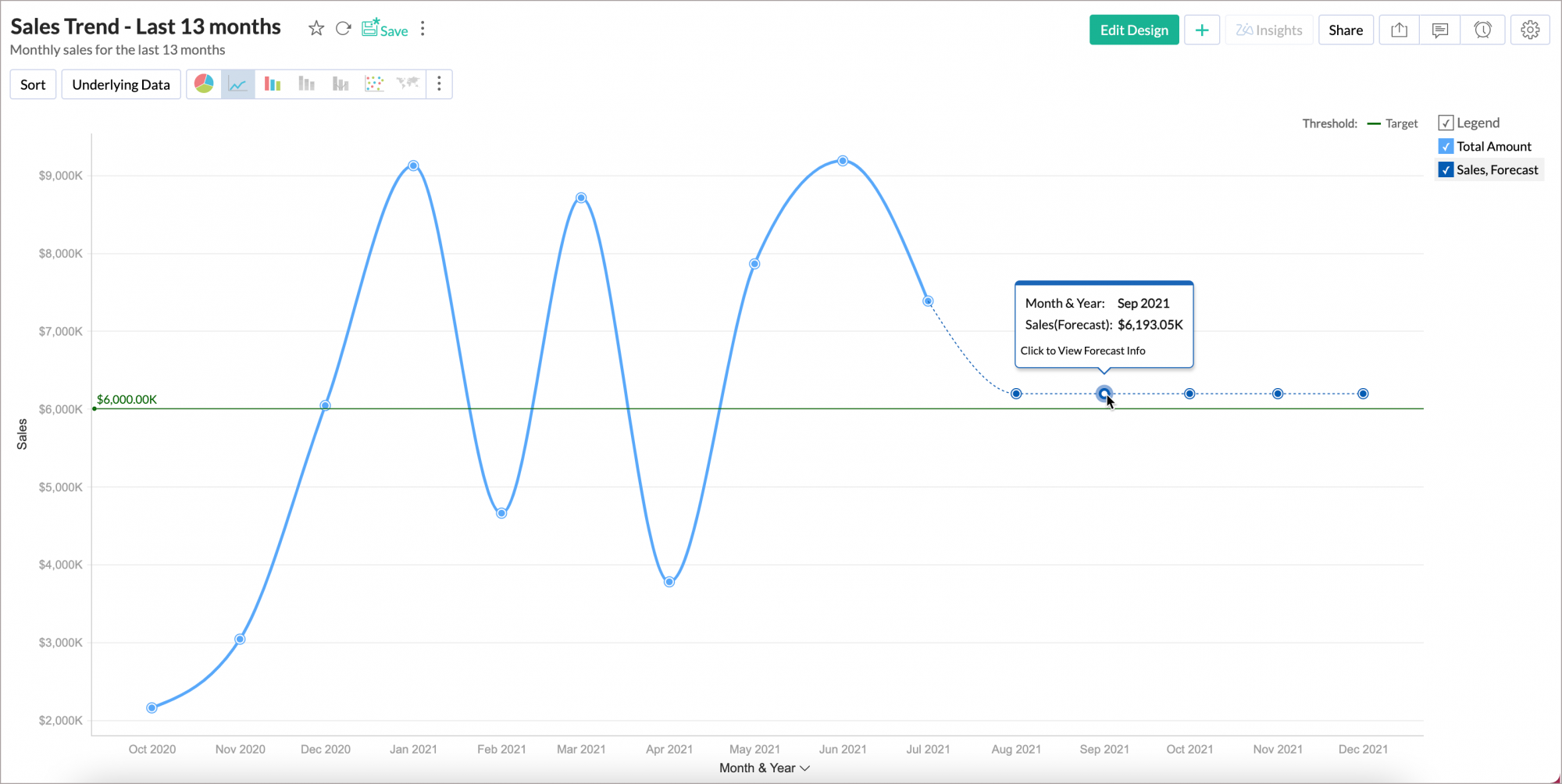• In Edit Design mode, click Forecast Legend and then select Forecast Info.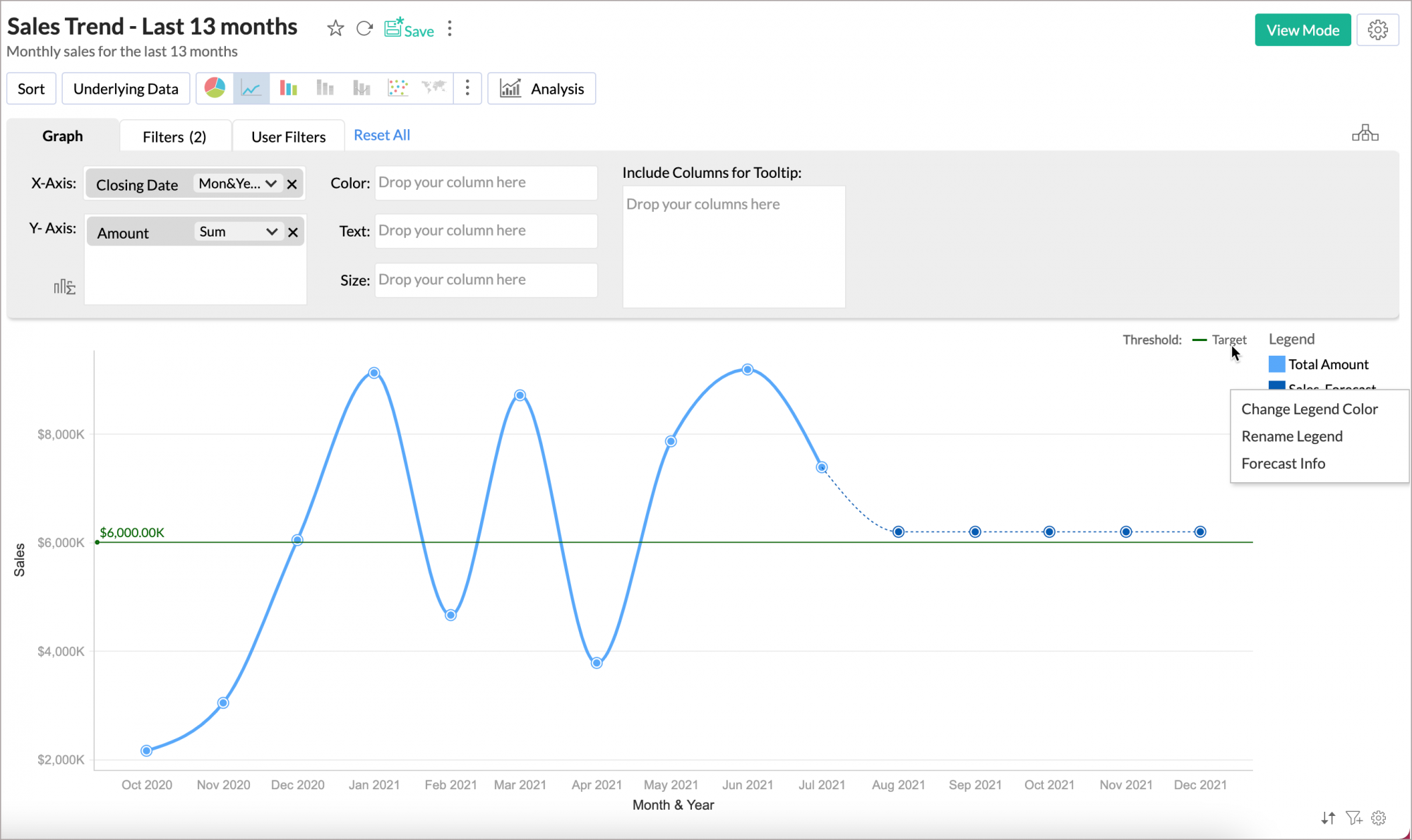• In the Forecast dialog, click the Forecast Info icon.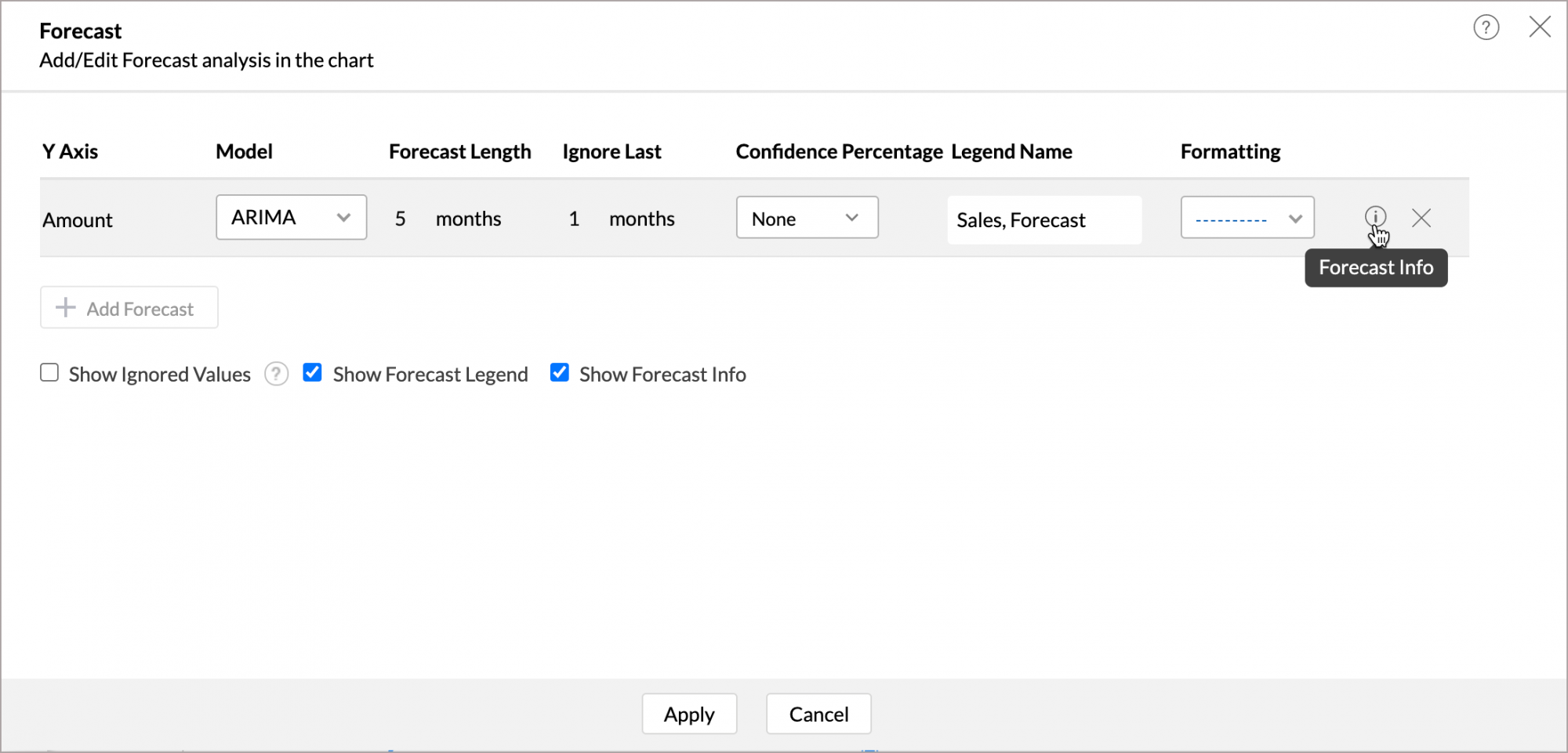The Forecast Model dialog will open displaying the following information.

General Information

The General Information section displays the following information:

• Training Period: This is the period of actual data based on which forecast is applied.
• Forecast Period: This is the forecasted period.

Model Information

The Model Information section displays the applied model details.

 Field Description Common Fields Forecast Model One of the four forecast models applied over the chart. {Forecast Model} Model The sub model that is applied over the chart. Frequency Frequency of the time series. Data Components Presence of Trend and Seasonality in the data. ARIMA Arima Coefficients This is the weight of past observations of the data. This will decay or go to zero since the current observation is more closely related to the most recent observations rather than to old observations.Note: Will be hidden when there is no autoRegressive, movingAverage , seasonalAutoRegressive & seasonalMovingAverage terms. i,e., when p=q=P=Q=0 STL Decomposed Values The actual data will be split into three components namely Trend, Seasonality and Residue and will be displayed. ETS This shows smoothing parameters for Level, Trend, and Seasonality. Regression R Square This indicates how better the model fits the actual data. Adjusted R Squared This ensures that the model does not overfit.  The model with the highest Adjusted R Squared will be applied over your data. F Statistic This shows how far the X value influences the trend. The higher the value the more it (X value) contributes to the trend. P Value This shows the probability of the estimator not contributing to the data. This indicates the significance of the Coefficients. The smaller the p-value, the more significant the model is.

Performance Indicators

The Performance Indicator shows the following information:

• RMSE: Root Mean Square Error shows how concentrated the data is around a trend.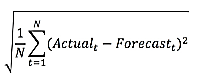• MAPE: The Mean Absolute Percentage Error is a measure of prediction accuracy of a forecasting method in statistics.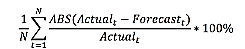• WMAPE: It's a variant of MAPE in which errors are weighted by values of actuals.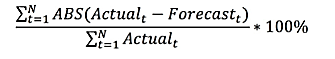• Quality: The Forecast quality is calculated based on LEP. Possible values are:
• GOOD
• ACCEPTABLE
• POOR
• AICC: This is a model to check the quality of the forecast.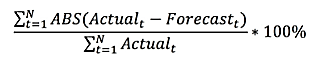• BIC: This is a model to check the quality of the forecast. Following is the formula.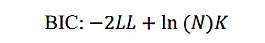Where,
LL -> the  model  log likelihood estimate,
K  ->  Number of parameters (e.g., 3 if (alpha, beta & gamma) are used)
n  ->  sample size

• LEPS: Linear Error in Probability Space is the mean absolute difference between the forecast cumulative distribution value (CDF) and the observation

## Troubleshooting Tips

### 1. I could not find "Forecast" option in chart settings. Why?

This could happen when the forecast constraints are not met. Please refer to the constraints specified.

### 2. Already configured forecasting settings are not available now. Why?

This could happen when the design of the chart has been modified, which does not match the forecast constraints.

### 3. I have configured forecasting for my chart. But it says, "Forecasting is disabled as the data is completely ignored". Why?

This could happen if you had set to ignore all the past data points from "Ignore Last" setting.

### 4. I have configured forecasting for my chart. But it says, "Forecasting is disabled as there is not enough data to identify pattern". Why?

This could happen when there is no sufficient data produced to forecasting engine to come up with forecasted data points.

### 5. I have configured forecasting for my chart. But it says, "Forecasting is disabled as there are more than 40% empty values". Why?

When the past data points provided to the forecasting engine has more null values, the forecasted points might be inaccurate. To avoid this, the forecasting engine will discard the process when the null values are more than 40% in the given data.

### 6. I have configured forecasting for my chart. But it says, "Column cannot be forecast as more than 5 data points is required". Why?

To produce an accurate forecast, the data points to be considered for forecasting should be more than 5 points. Try changing the time series in X-axis to a more granular function which may result in more data points. For example, If the existing time series is Year, then change to Month & Year.